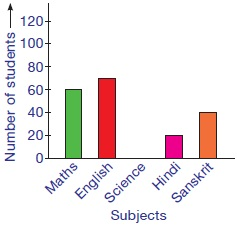# User ForumSubject :IMO    Class : Class 4

The given bar graph shows the subjects liked by 225 students of class 4.Find the total number of students who liked Science and English.

A 105
B 70
C 35
D 115

Question is confusing. The science bar is blank, so it can be considered as 0. However if the sum of other subject is deducted from the total 225, science comes to 35.The correct answer is considering science value as 35.

## Ans 1:

Class : Class 5
There is no figure given for science student thus the answer is B.

## Ans 2:

Class : Class 4
Answer should be A not B.

## Ans 3:

Class : Class 5
answer should be b not a

Class : Class 4

Class : Class 4
A

Class : Class 6
A

## Ans 7:

Class : Class 6
The question is wrong because the total is 225 students and to find number of students who like science we will subtract the total of maths,english ,hindi and sanskrit with 225 and add the answer to students who like english .So the answer came as 100

Class : Class 4

## Ans 9:

Class : Class 4
its not a direct question....

## Ans 10:

Class : Class 4
thats true. we are more intelligent than them

## Ans 11:

Class : Class 8
answer should be B not A

## Ans 12:

Class : Class 7
but the given data is wrong and ans a not b

Class : Class 7

Class : Class 3

## Ans 15:

Class : Class 4
The answer should be A. There is no value had given for science. Without that the total is 190. If we calculate the marks scored science out of 225, then the mark in science has to be 35. Hence the total has to 105, which is answer A

Class : Class 7

## Ans 17:

Class : Class 5
a is the answer because there are 225 students and without science it is only 190

## Ans 18:

Class : Class 3
answer should be B not A

## Ans 19:

Class : Class 6
Yes it should be A as without science the number of students would be 190. Here the total number of students is 225

Class : Class 6

## Ans 21:

Class : Class 6
No! The answer is A. 60 70 20 40 = 190 which is NOT 225.Science = 35, English = 70 so answer is 105(A).

Class : Class 6
A

## Ans 23:

Class : Class 4
A is the correct answer as without science there will be 190 students

Class : Class 4
a

Class : Class 5

Class : Class 8

## Ans 27:

Class : Class 4
Answer should be B not A

Class : Class 5

## Ans 29:

Class : Class 4
ANSWER IS A. Total number of students is 225. So science has to be 35. Its a glitch question!

Class : Class 7